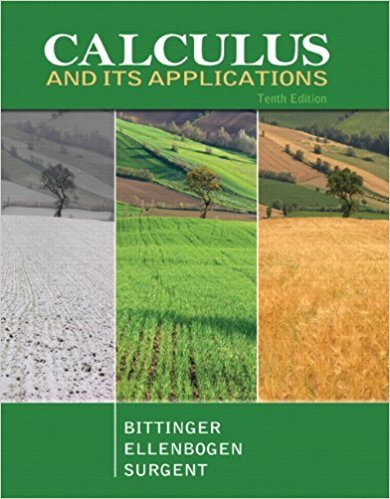×
×

# Solutions for Chapter 3: Exponential and Logarithmic Functions## Full solutions for Calculus and Its Applications | 10th Edition

ISBN: 9780321694331Solutions for Chapter 3: Exponential and Logarithmic Functions

Solutions for Chapter 3
4 5 0 258 Reviews
13
4
##### ISBN: 9780321694331

Chapter 3: Exponential and Logarithmic Functions includes 53 full step-by-step solutions. This expansive textbook survival guide covers the following chapters and their solutions. Calculus and Its Applications was written by and is associated to the ISBN: 9780321694331. Since 53 problems in chapter 3: Exponential and Logarithmic Functions have been answered, more than 24087 students have viewed full step-by-step solutions from this chapter. This textbook survival guide was created for the textbook: Calculus and Its Applications, edition: 10.

Key Calculus Terms and definitions covered in this textbook
• Annuity

A sequence of equal periodic payments.

• Back-to-back stemplot

A stemplot with leaves on either side used to compare two distributions.

• Confounding variable

A third variable that affects either of two variables being studied, making inferences about causation unreliable

• Constraints

See Linear programming problem.

• Coordinate plane

See Cartesian coordinate system.

• Coordinate(s) of a point

The number associated with a point on a number line, or the ordered pair associated with a point in the Cartesian coordinate plane, or the ordered triple associated with a point in the Cartesian three-dimensional space

• Definite integral

The definite integral of the function ƒ over [a,b] is Lbaƒ(x) dx = limn: q ani=1 ƒ(xi) ¢x provided the limit of the Riemann sums exists

• Elementary row operations

The following three row operations: Multiply all elements of a row by a nonzero constant; interchange two rows; and add a multiple of one row to another row

• Exponential growth function

Growth modeled by ƒ(x) = a ? b a > 0, b > 1 .

• Factor

In algebra, a quantity being multiplied in a product. In statistics, a potential explanatory variable under study in an experiment, .

See Polynomial function in x.

• Logistic regression

A procedure for fitting a logistic curve to a set of data

• Major axis

The line segment through the foci of an ellipse with endpoints on the ellipse

• Matrix, m x n

A rectangular array of m rows and n columns of real numbers

A function that can be written in the form ƒ(x) = ax 2 + bx + c, where a, b, and c are real numbers, and a ? 0.

• Quotient of functions

a ƒ g b(x) = ƒ(x) g(x) , g(x) ? 0

• Random variable

A function that assigns real-number values to the outcomes in a sample space.

• Re-expression of data

A transformation of a data set.

• Reflexive property of equality

a = a

• Third quartile

See Quartile.

×